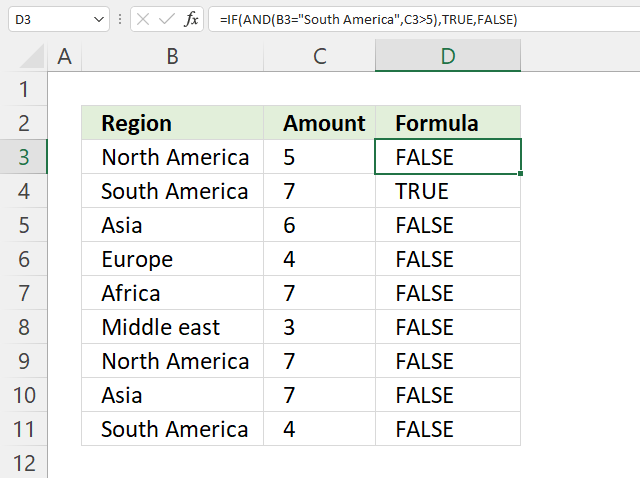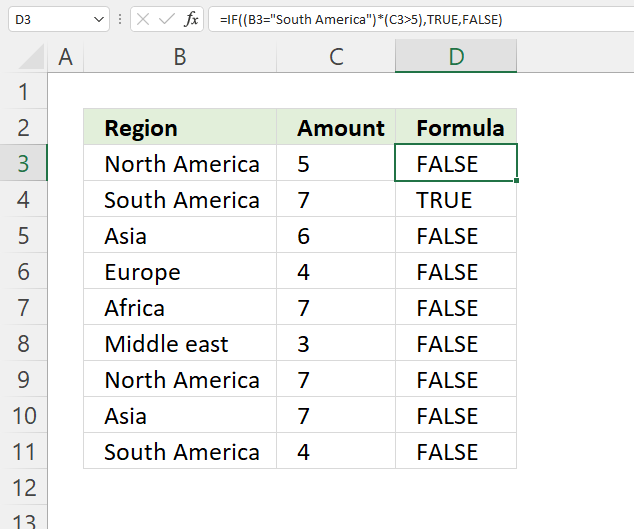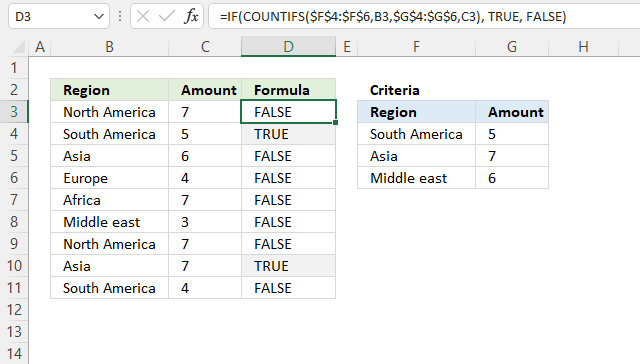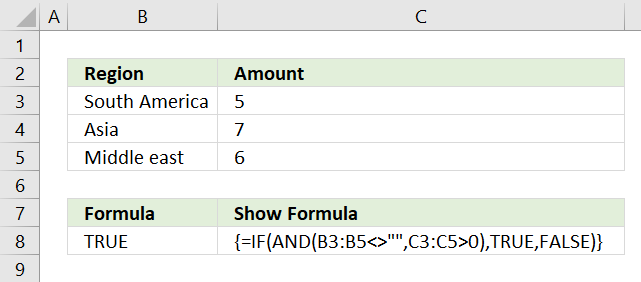Author: Oscar Cronquist Article last updated on October 14, 2021The AND function allows you to have multiple conditions in an IF function, you can have up to 254 arguments. An argument is an input value given to a function. You construct a logical expression that you use as an argument in the AND function.

## 1. IF with AND function - two logical expressionsFormula in cell D3:

=IF(AND(B3="South America", C3>5), TRUE, FALSE)

### 1.1 Explaining formula in cell D3

The IF function above checks two conditions, the "Region" value must match a text string and the "Amount" value must be larger than a number. If both conditions return TRUE the AND function returns TRUE.

IF REGION = value AND Amount > number then TRUE Else FALSE

In other words, all logical tests in each argument in the AND function must return TRUE for the AND function to return TRUE. The AND function returns FALSE if at least one argument returns FALSE.

#### Step 1 - Check if value equals condition

The equal sign compares value to value, it returns a boolean value TRUE or FALSE.

B3="South America"

becomes

"North America"="South America"

and returns FALSE.

#### Step 2 - Check if the number is greater than the condition

The larger than character lets you check if a value is larger than another value, it also returns a boolean value TRUE or FALSE.

C3>5

becomes

5>5

and returns FALSE. Five is not larger than five.

#### Step 3 - Evaluate AND function

The AND function returns a boolean value TRUE or FALSE if all arguments evaluate to TRUE or the numerical equivalent which is one.

AND(B3="South America", C3>5)

becomes

AND(FALSE, FALSE)

and returns FALSE.

#### Step 4 - Evaluate IF function

The IF function returns one value if the logical test is TRUE and another value if the logical test is FALSE.

IF(logical_test, [value_if_true], [value_if_false])

IF(AND(B3="South America", C3>5), TRUE, FALSE)

becomes

IF(FALSE, TRUE, FALSE)

and returns FALSE.

### 1.2 Alternative formulaYou can shorten the formula somewhat by enclosing each logical expression with parentheses and then multiply the conditions.

Formula in cell D4:

=IF((B4="South America")*(C4>5), TRUE, FALSE)

#### Step 1 - Check the first condition

The first logical expression in cell B4 is (B4="South America") and it returns TRUE.

B4="South America"

becomes

"South America"="South America"

and returns TRUE.

#### Step 2 - Check the second condition

The second expression (C4>5) also returns TRUE.

C4>5

becomes

7>5

and returns TRUE.

#### Step 3 - Multiply boolean values

(B4="South America")*(C4>5)

becomes

TRUE * TRUE

equals 1. TRUE multiplied by TRUE is 1. TRUE is the same thing as 1 and FALSE is 0 (zero). If you multiply boolean values the outcome is always 0 (zero) or 1.

#### Step 4 - Evaluate IF function

The IF function returns one value if the logical test is TRUE and another value if the logical test is FALSE.

IF(logical_test, [value_if_true], [value_if_false])

IF((B4="South America")*(C4>5), TRUE, FALSE)

becomes

IF(TRUE, TRUE, FALSE)

and returns TRUE.

## 2. IF with AND function - multiple pairs of criteriaThe formula in cell D3 checks if any of the criteria pairs in cell range F4:G6 matches cell B3 and C3 respectively.

Formula in cell D3:

=IF(COUNTIFS(\$F\$4:\$F\$6,B3,\$G\$4:\$G\$6,C3), TRUE, FALSE)

### 2.1 Explaining formula in cell D3

#### Step 1 - Check multiple conditions

The COUNTIFS function calculates the number of cells across multiple ranges that equals all given conditions.

It allows you to use up to 254 arguments or 127 criteria pairs.

Excel Function Syntax
COUNTIFS(criteria_range1, criteria1, [criteria_range2, criteria2]…)

COUNTIFS(\$F\$4:\$F\$6,B3,\$G\$4:\$G\$6,C3)

becomes

COUNTIFS({"South America"; "Asia"; "Middle east"}, "North America", {5; 7; 6}, 7)

and returns 0 (zero).

#### Step 2 - Evaluate IF function

The IF function returns one value if the logical test is TRUE and another value if the logical test is FALSE.

IF(logical_test, [value_if_true], [value_if_false])

IF(COUNTIFS(\$F\$4:\$F\$6,B3,\$G\$4:\$G\$6,C3), TRUE, FALSE)

becomes

IF(0, TRUE, FALSE)

and returns FALSE in cell D3. 0 (zero) is the numerical equivalent to FALSE.Cell D4 returns TRUE, both cells B4 and C4 match cells F4 and G4.

## 3. Using arrays in the AND functionThe array formula above lets you check if all values in cell range B3;B5 are not empty and if all numbers in C3:C5 are above 0 (zero). It returns TRUE if all conditions are TRUE.

Formula in cell B8:

=IF(AND(B3:B5<>"",C3:C5>0), TRUE, FALSE)

There are many conditions in the above formula and the array formula lets you do this without problems.

To enter an array formula press and hold CTRL + SHIFT simultaneously, then press Enter once. Release all keys.

The formula bar now shows the formula enclosed with curly brackets telling you that you entered the formula successfully. Don't enter the curly brackets yourself.

### 3.1 Explaining formula in cell B8

#### Step 1 - Check if all values in the array are non-empty

The less than and larger than characters combined lets you check if a value is not equal to another value. The result is a boolean value TRUE or FALSE.

This can be performed to an array of values as well, it returns as many boolean values as there are values in the array.

B3:B5<>""

becomes

{"South America";"Asia";"Middle east"}<>""

and returns {TRUE; TRUE; TRUE}. All values in the cell range are not equal to nothing "".

#### Step 2 - Check if values are larger than zero

The larger than character lets you check if a value is larger than another value, it also returns a boolean value TRUE or FALSE.

C3:C5>0

becomes

{5; 7; 6}>0

and returns {TRUE; TRUE; TRUE}.

#### Step 3 - Check if all values are TRUE

The AND function returns a boolean value TRUE or FALSE if all arguments evaluate to TRUE or the numerical equivalent which is one.

AND(B3:B5<>"",C3:C5>0)

becomes

AND({TRUE; TRUE; TRUE}, {TRUE; TRUE; TRUE})

and returns TRUE.

#### Step 4 - Evaluate IF function

The IF function returns one value if the logical test is TRUE and another value if the logical test is FALSE.

IF(logical_test, [value_if_true], [value_if_false])

IF(AND(B3:B5<>"",C3:C5>0), TRUE, FALSE)

becomes

IF(TRUE, TRUE, FALSE)

and returns TRUE.